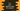# C++ program to print the rightmost digit of a number## C++ program to print the rightmost digit of a number:

In this post, we will learn how to print the rightmost digit of a number in C++. We will write one C++ program that will take one number as input from the user and print out the rightmost digit on console.

### C++ program:

Let’s take a look at the program below:

``````#include <iostream>
using namespace std;

int main()
{
int number;
cout << "Enter a number : " << endl;
cin >> number;

cout << "Last digit of the number : " << number % 10 << endl;

return 0;
}``````

Here, we are using modulo % to find the last number. x % y returns the remainder of x/y. So, if we use x % 10, it will return the remainder of x/10, which is the rightmost digit of a number.

### Sample output:

It will print output as like below:

``````Enter a number :
12349
Last digit of the number : 9

Enter a number :
123456
Last digit of the number : 6``````

### Last digit for a negative number:

The above method works only for positive numbers. If we want for negative numbers as well, we need to use it with absolute value of the number. We can use abs() to find the absolute value for a number.

Below is the program:

``````#include <iostream>
using namespace std;

int main()
{
int number;
cout << "Enter a number : " << endl;
cin >> number;

cout << "Last digit of the number : " << abs(number) % 10 << endl;

return 0;
}``````

It will give output as like below:

``````Enter a number :
-2378
Last digit of the number : 8

Enter a number :
1238
Last digit of the number : 8

Enter a number :
0090990
Last digit of the number : 0``````